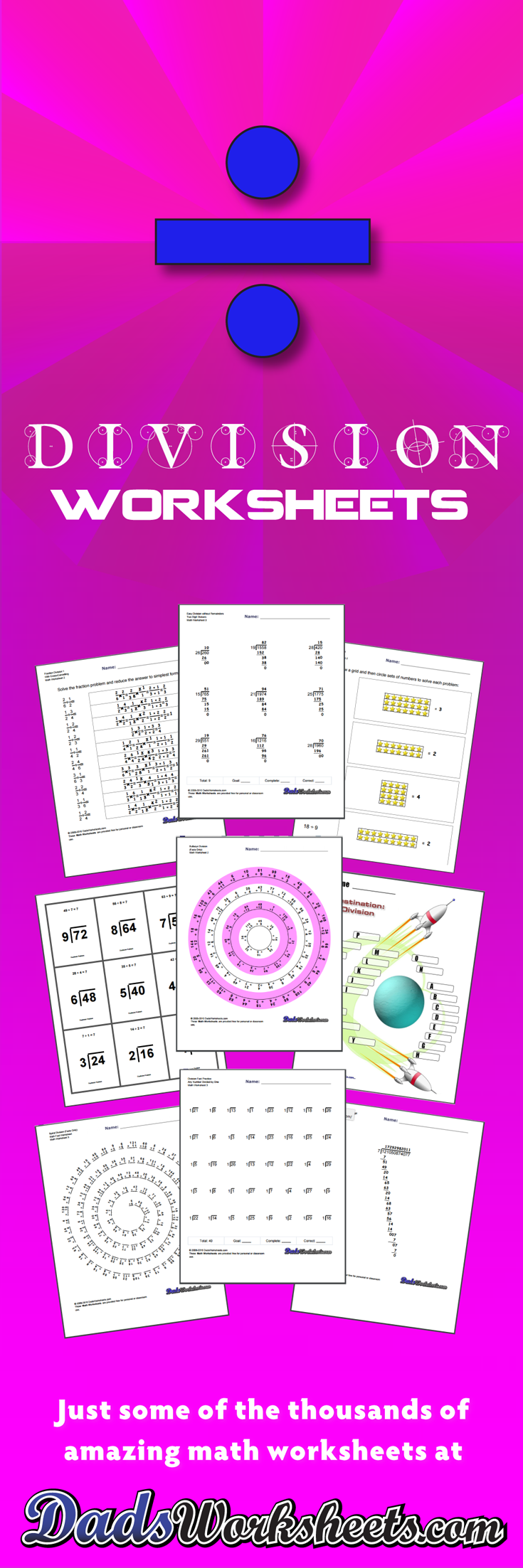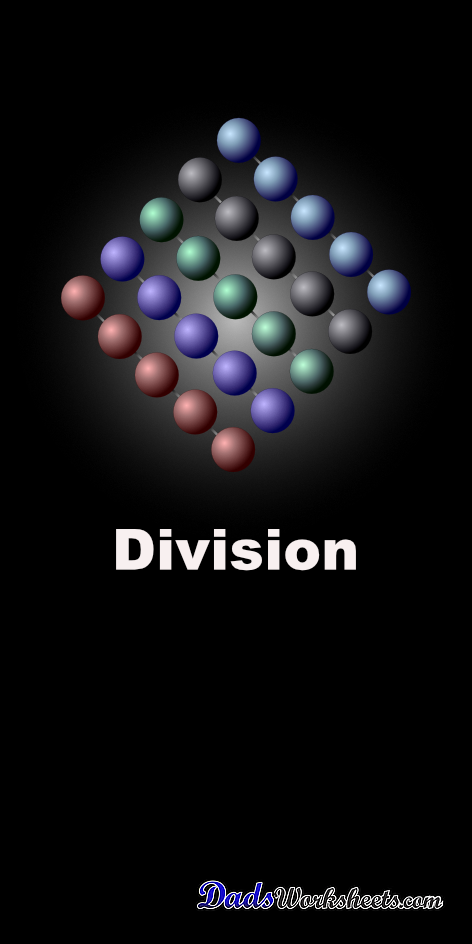# Division Worksheets

These division worksheets start with basic timed math fact drills in formats for one minute and two minute tests. There are variations that include problems with and without remainders to build the skill set needed for long division, and there is focused division practice for roots and powers of ten as well. If you're not worn out after all that dividing, please try out the unique spiral and bullseye division fact worksheets to get a completely different spin on your math fact practice. And, if I missed anything division-related, please let me know!

## Division Facts Worksheets

### 40 Division Worksheets

These are basic practice division worksheets designed to work as one minute timed tests or exercise for simple division problems. Each set of division worksheets focuses on a specific set of division problems with a common divisor.

Division Facts Worksheets

## One Minute Timed Division Worksheets

### 96 Division Worksheets

The practice division worksheets below are similar to the RocketMath, Mad Math Minutes or Mastering Math Facts division worksheets used at many schools. These are typically one minute, timed tests (follow the 'Timer' link off the home page.) Note: These sheets include problems with divisors larger than the dividend starting at Level G, which is normal for these programs. If you want problems with only 'real' division problems, see the 'Facts Only' series of worksheets further down on this page.

SpaceshipMath Division Worksheets

## Two Minute Division Worksheets

### 56 Division Worksheets

80 problem division worksheets similar to RocketMath tests designed for two minute drills. Each of these division worksheets combines two levels from one minute tests, introducing four to five new division problems on each worksheet.

Two Minute Division Worksheets

## Extra Spaceship Math Division Worksheets

### 56 Division Worksheets

These worksheets are extensions to the Spaceship Math and Rocket Math division worksheets for additional larger division problems.

Extra Spaceship Math Division Worksheets

## SpaceshipMath Division Worksheets - Just Whole Facts

### 124 Division Worksheets

The Spaceship/RocketMath division worksheets above include division problems where the divisor is less than the dividend, and the quotient is always zero. If you are looking for division worksheets with just the whole answer division facts, the worksheet set below has you covered!'

SpaceshipMath Division Worksheets - Just Whole Facts

## Whole Facts Division Worksheets - Two Minute Tests

### 20 Division Worksheets

Extensions to SpaceshipMath and RocketMath division worksheets for additional division facts, 80 problem two minute timed division tests.

Whole Facts Division Worksheets - Two Minute Tests

## Circle Division Facts Worksheets

### 32 Division Worksheets

These division worksheets emphasize groups of facts with common divididends. Try the variations with all facts, or print the worksheets that focus only on specific families of division facts that need more practice!

Division Fact Circles

## Spiral Division Worksheets

### 8 Division Worksheets

Tired of the same old division fact worksheets with rows and rows of problems? Time to try something unique. These worksheets present the division facts in a spiral layout that provides fun a twist on memorizing the fact tables. They use the same fact layouts as the spaceship math sheets above, so try the first two sets worksheets if you are looking for the full set of multiplication facts or practice without the easier problems, or look at the others for an incremental approach to learning the facts.

Spiral Division Worksheets

## Bullseye Division Worksheets

### 8 Division Worksheets

Get on target with those division math facts! These fun worksheets provide some additional kinesthetics over and above your usual math fact practice. Hopefully you will find these division worksheets are spot-on when you need a change of pace!

Bullseye Division Worksheets

## SpaceshipMath Division Worksheets - Focus on Remainders

### 96 Division Worksheets

Mastered the RocketMath or SpaceShip Math division worksheets? Have a basic understanding of remainders? This series of division worksheets will build instant recognition of common remainder results!

SpaceshipMath Division Worksheets - Focus on Remainders

## SpaceshipMath Division Worksheets - All with Remainders

### 40 Division Worksheets

Division worksheet drills with all of the facts, including remainders!

SpaceshipMath Division Worksheets - All with Remainders

## Division Worksheets with Progressive Remainders

### 20 Division Worksheets

These division worksheets build recognition of base remainders for small dividends. They are great skill builders to follow the basic division worksheets that focus on simple facts.

Division Worksheets with Progressive Remainders

## Doubles Plus One Division Worksheets

### 28 Division Worksheets

Division worksheets for doubles plus one for all doubles less than 100 (Worksheets are progressive).

Doubles Plus One Division Worksheets

## Division Worksheets for Roots

### 8 Division Worksheets

These division worksheets build up to knowledge of square and cube roots.

Division Worksheets for Roots

## Mixed Multiplication and Division Worksheets

### 8 Division Worksheets

This collection of multiplication and division worksheets can be used for timed practice once both multiplication and division problems have been mastered. These worksheets are a great way to help reinforce the inverse relationship between multiplication and division math facts.

Mixed Multiplication and Division Worksheets

## Factors of Ten Division Worksheets

### 12 Division Worksheets

Division worksheets with factors of 10.

Factors of Ten Division Worksheets

## Powers of Two Division Worksheets

### 24 Division Worksheets

Get your budding software developer ready by practicing the binary progression using division worksheets. For non-programmers, introduces an interesting pattern of values that makes for great reinforcement of division concepts.

Powers of Two Division Worksheets

## Division Worksheets

Once the other three math operations are mastered, the final mountain to climb is division! This page focuses on the learning the core division problems, but it does include division worksheets that introduce remainders to simple problems that should be capable of being performed mentally. Practicing division with simple remainders will provide a solid base for learning long division. When you students are ready, long division worksheets are available at the links below, along with other division worksheets that introduce this math operation in different ways: